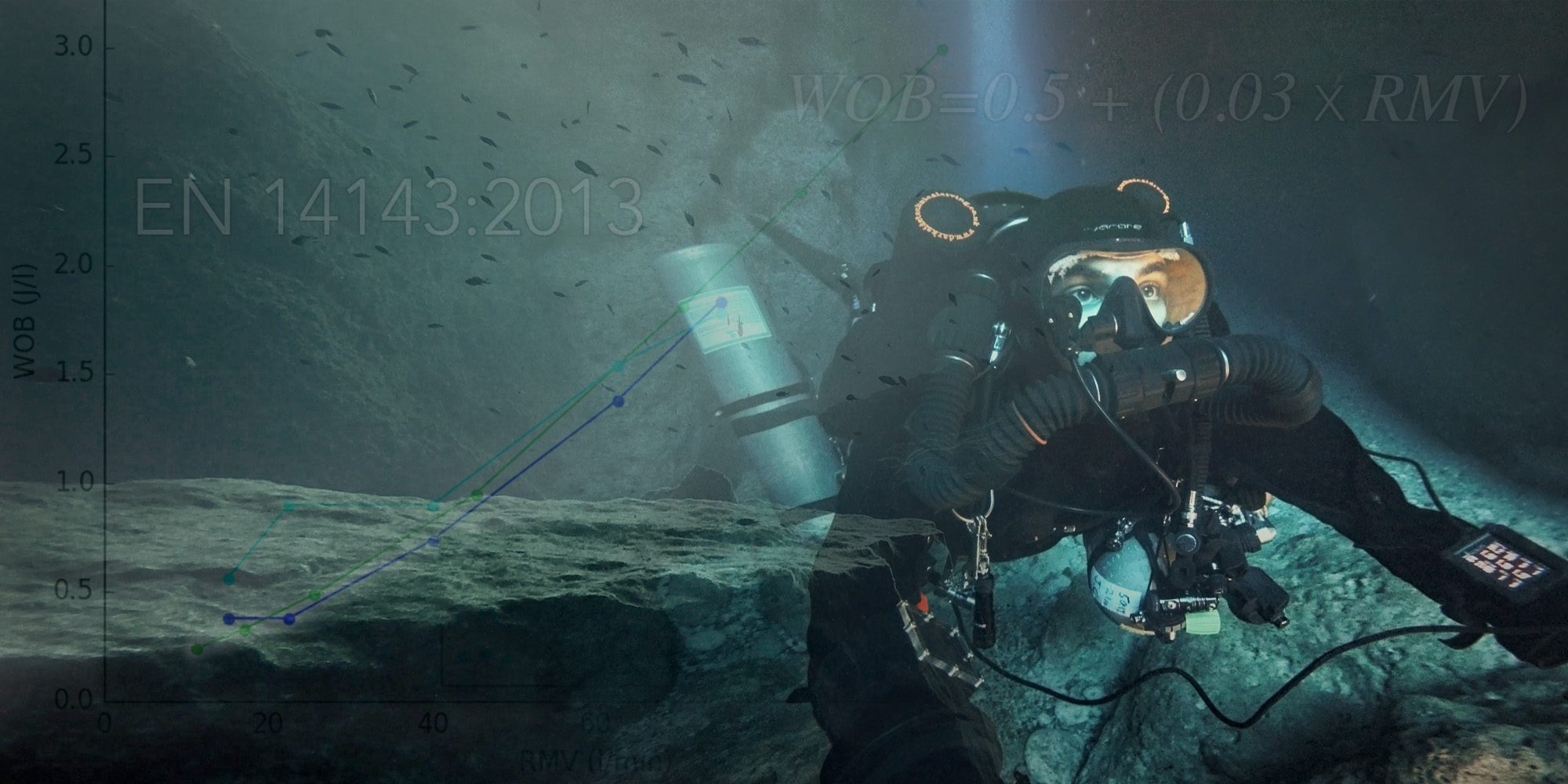# A Comparison of CE Test Data for two Closed Circuit Rebreathers

This post provides a comparison of some available CE-testing data on the breathing characteristics of two rebreathers currently on the market: the JJ-CCR and X-CCR.  The comparison was carried out mostly for my own interest, but I decided to post it here in case it might be of interest to others as well.  (The code was written in Python 3.5.2 and all plots produced with matplotlib.)

Firstly, a few words of note.

The configuration of the two rebreathers is different.  To mention but two examples: the JJ uses an axial canister whereas the X-CCR uses a radial one, and the JJ is fitted with a DSV whereas the X-CCR uses a BOV.  The available CE test data are for the units set up as per the above respective configurations.

The CE testing documentation for the JJ states that tests were carried out at a pitch angle of 74o (with the exception of the testing of hydrostatic imbalance vs. pitch angle, of course, because in that case the pitch angle needs to be varied since that is precisely what is being tested.  Tests of the X-CCR were presented at pitch angles of 0o (i.e. “in trim”) and 90o (i.e. vertically upright).  The reader should note that in some cases, a perfect, direct comparison of the two units is not possible due to the different pitch angles at which data were collected for each respective unit.  This should be borne in mind throughout.

Tests at 100m using trimix were carried out using a similar (but slightly different) mix for each unit.  In the case of the JJ it was 11/65 whereas for the X-CCR a (slightly less dense) mix of 10/70 was used.

In each of the below figures, I have included the EN14143 threshold in red.  You do not want to approach or (worse) exceed the threshold.  Exceeding the established threshold compromises the ability to obtain CE certification.  The further away the data lie from this threshold, the better.

WORK OF BREATHING

As the name implies, work of breathing denotes the amount of work required to breathe.  When we draw in a breath, our muscles cause our lungs to expand, and if this process encounters any resistance, the work required to expand the lungs will be higher.

Work of breathing (WOB) is described via the simple equation: WOB=0.5+0.03×RMV, where RMV stands for Respiratory Minute Volume.  The latter simply means the volume of gas inhaled (or exhaled) per minute.  The higher the RMV, the higher the WOB.

Basing on the available data, both at 40m (using air) and 100m (using trimix), the WOB for the JJ and the X-CCR is virtually identical, with the JJ’s figures (at 74o) running approximately between the X-CCR’s 0o and 90o tests.

If the performance between the two units were identical, this would kind of be expected, since 74o sits between the other two angles (0o and 90o).  However, it is very important to note that one cannot comment further basing on this plot; a perfect comparison cannot be drawn from this plot since the pitch angles at which the tests for the two respective units were carried out are different.  A more direct comparison can be made when considering the next test: hydrostatic imbalance.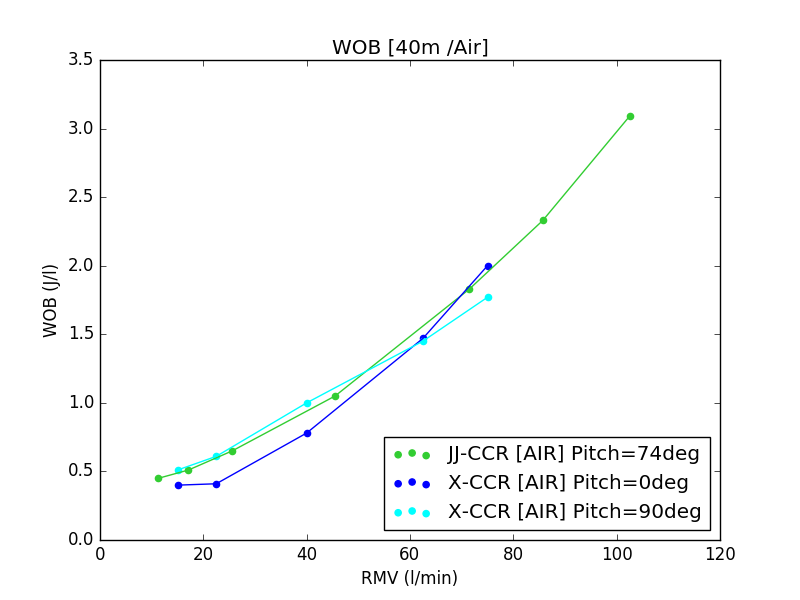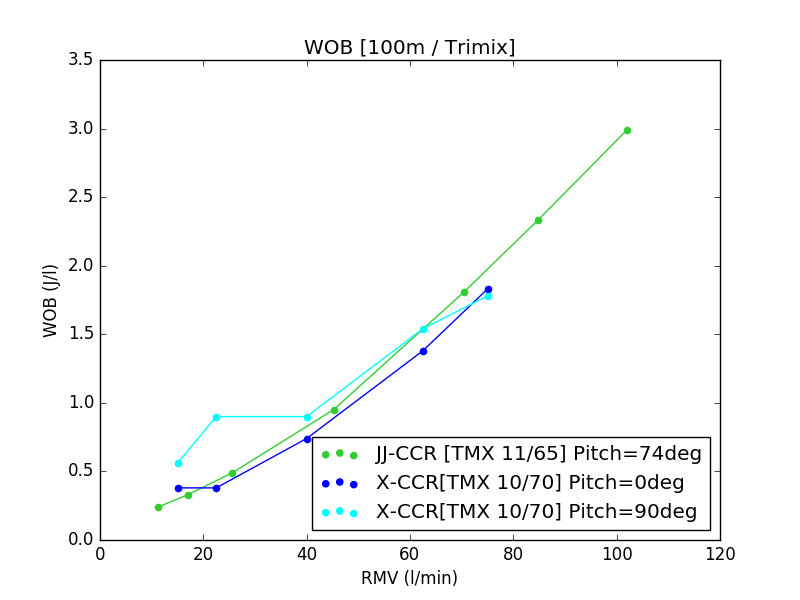HYDROSTATIC IMBALANCE

The counterlungs (CLs) are rarely located at the exact same depth as the diver’s lungs; this means that there will be a difference between the ambient pressure acting upon the CLs and that acting upon the diver’s lungs.  This results in the diver breathing at lower or higher volumes, which they will attempt to resist via muscle tensioning.

For example, in the case of a back-mounted configuration, the CLs lie atop the diver’s back, which means that when the diver is swimming in trim, the ambient pressure on the CLs will be lower than that acting upon the diver’s lungs, simply because the CLs are located higher up in the water column (i.e. they are positioned at a shallower depth).  As a result, drawing in a breath will encounter some resistance (negative imbalance) such that breathing is performed at lower volume and inhalation feels difficult.

On the other hand, for a chest-mounted configuration, the CLs lie at greater depth, so the pressure on them is higher than on the diver’s lungs.  In this case, the inverse occurs: drawing in a breath will feel easier (as it is easier for gas to move from higher pressure to lower pressure) and breathing is performed at higher volume, but exhalation feels more difficult (as this time round the diver is pushing gas out against a pressure gradient).

The hydrostatic imbalance test measures this difference in pressure, and the test is typically carried out with respect to the suprasternal notch. The CE test involves two test cases:

(1) Maintaining the same roll angle but varying the pitch angle.
(2) Maintaining the same pitch angle but varying the roll angle.

• Part 1:  Varying Pitch Angle

This test maintains a fixed roll angle of 0o whilst varying the pitch angle.

The JJ shows better performance than the X-CCR between about -45o and 90o, i.e. in the range that is arguably most commonly encountered by divers.   The JJ has a single restriction at -90o if the ADV is left open.  (Note that the reason for this restriction is simply that the ADV activates at -90o to increase lung volume.*)  JJ carried out a further test with the ADV closed (optimised at a pitch angle of 45o), in which case the result moves back within threshold (shown in the plot with a dotted line).

*In practical terms, this restriction is a moot point as (1) divers will rarely be diving inverted, with their head upside down, and (2) if they do, they have the possibility of using the flow-stop to shut off the ADV and use the diluent MAV to add diluent as required.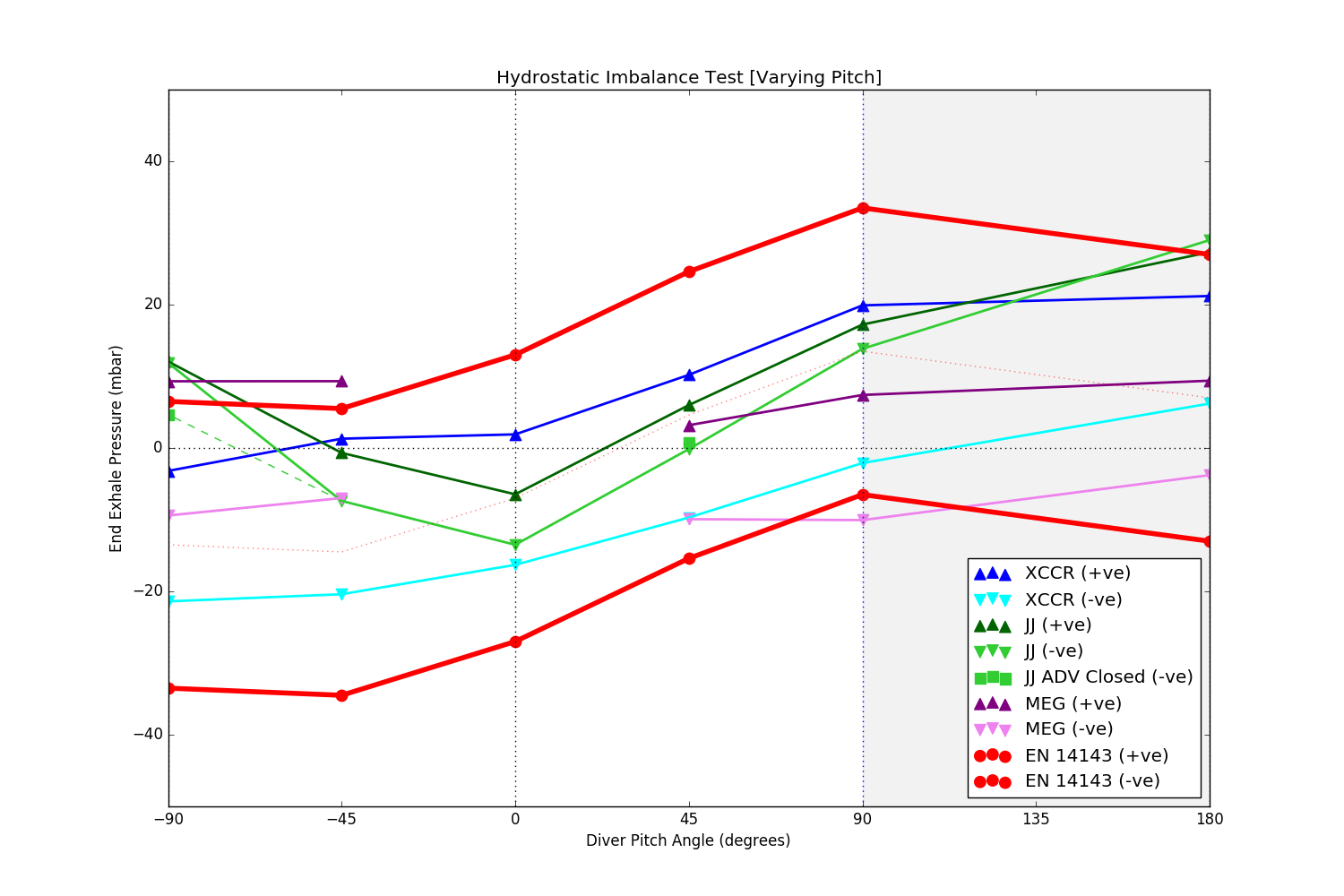• Part 2:  Varying Roll Angle

This test maintains a fixed pitch angle of 0o whilst varying the roll angle.  Here, throughout virtually the entire range of roll angles, the JJ performs better than the X-CCR, but both units are within EN14143 specs.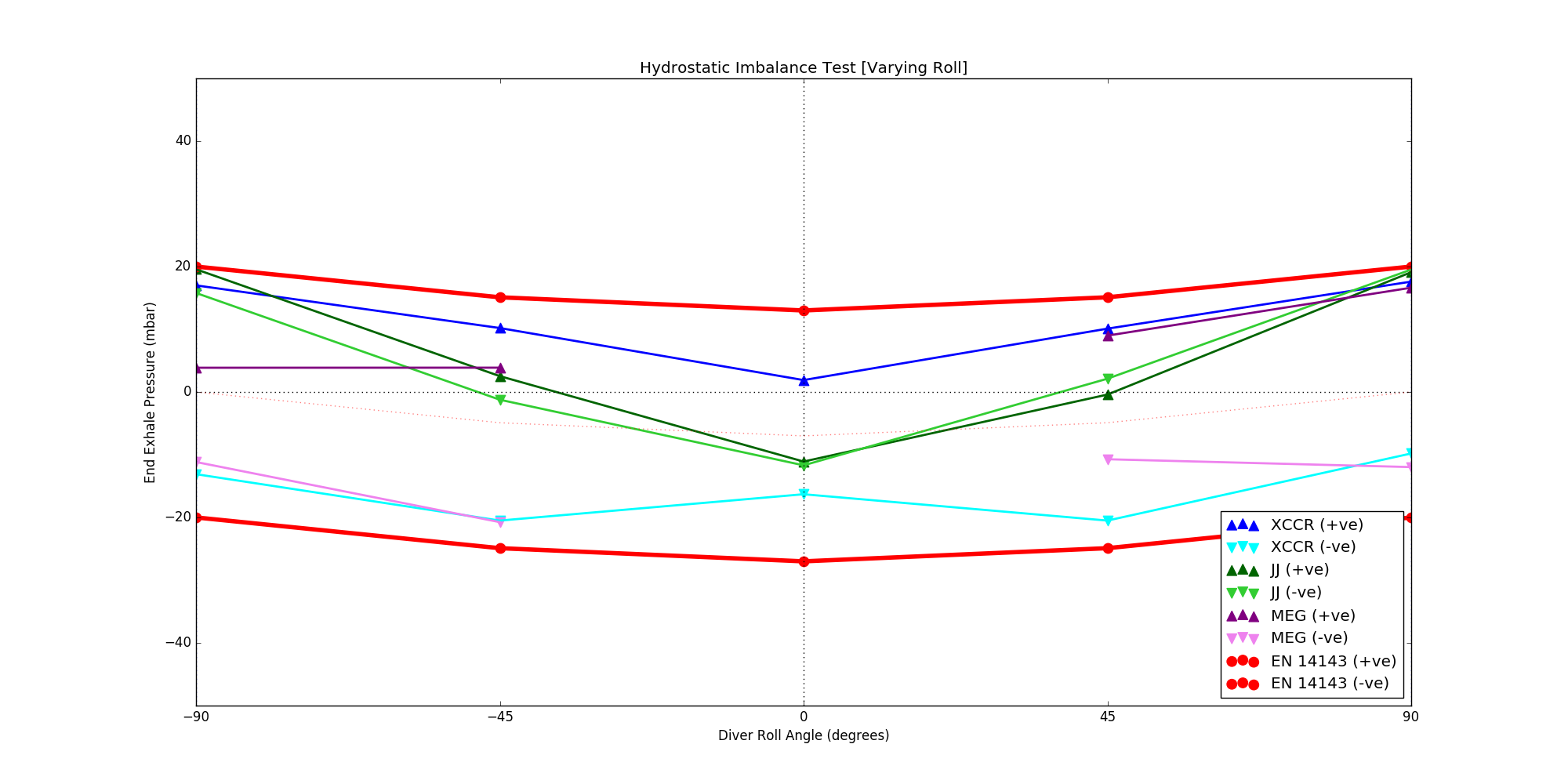For the interested reader, note that there is more data available for the JJ, as their test was carried out at a large number of pitch and roll angles, i.e. a more continuous range of angles.  These additional data points are shown on a graph that can be found on page 13 of the CE documentation of the JJ.

ELASTANCE

Elastance describes the resistance of an inflatable vessel of some sort (e.g. lungs) to expand upon applying a force.  If it takes a lot of force to make it expand, then it has high elastance, and vice versa.  Conversely, elastance denotes the tendency of said vessel to recoil back to its original volume when some force that had previously been acting upon it is removed.

• 40m (Air)

At pitch angle=0o, the X-CCR performs slightly better than the JJ (pitch angle=74o).
At pitch angle=90o, the X-CCR performs much worse than the JJ (pitch angle=74o) and exceeds the EN14143 threshold.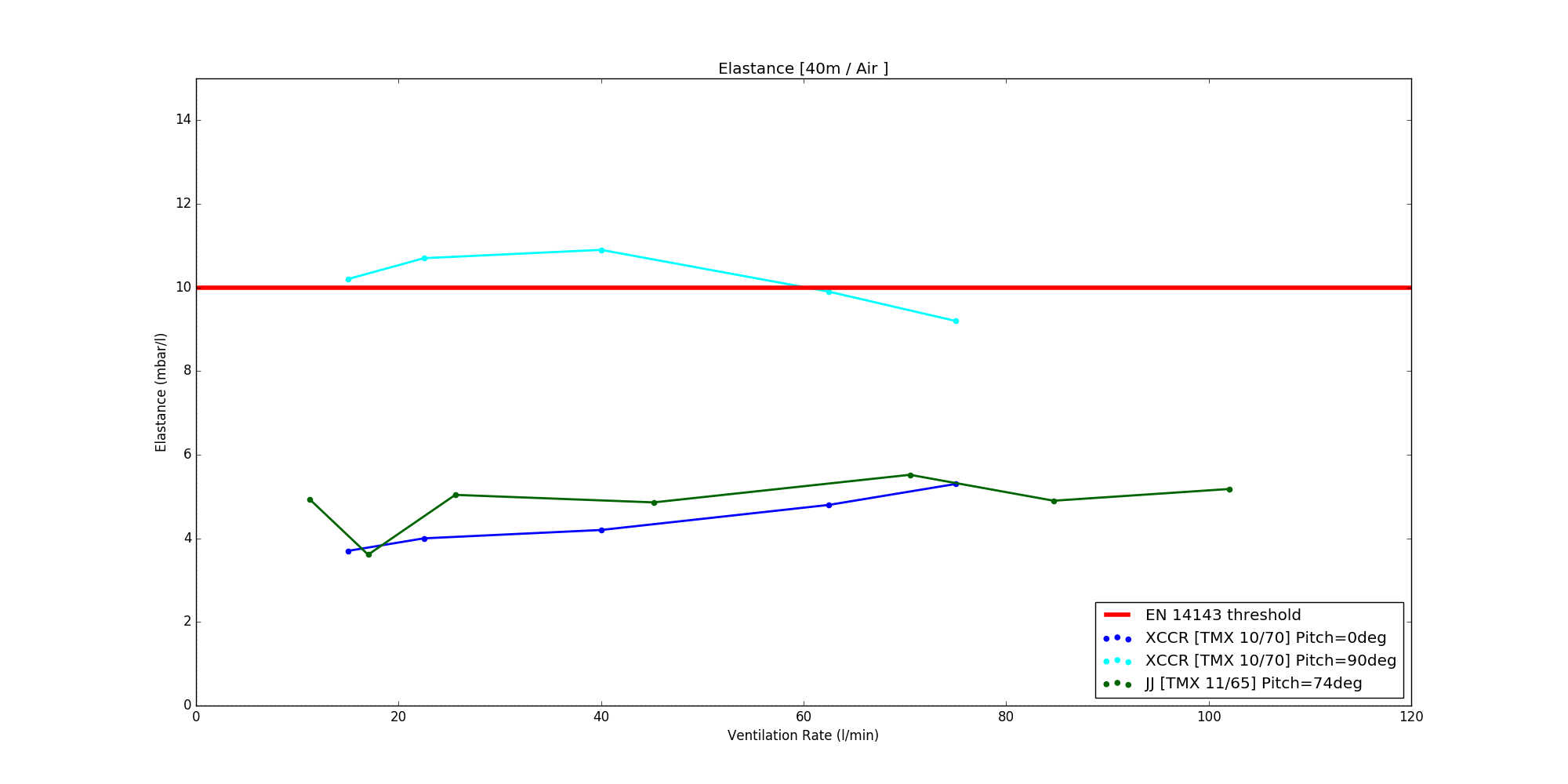• 100m (Trimix):

At pitch angle=0o, the X-CCR performs slightly better than the JJ (pitch angle=74o).
At pitch angle=90o, the X-CCR performs quite worse than the JJ (pitch angle=74o) but still sits within the EN14143 threshold.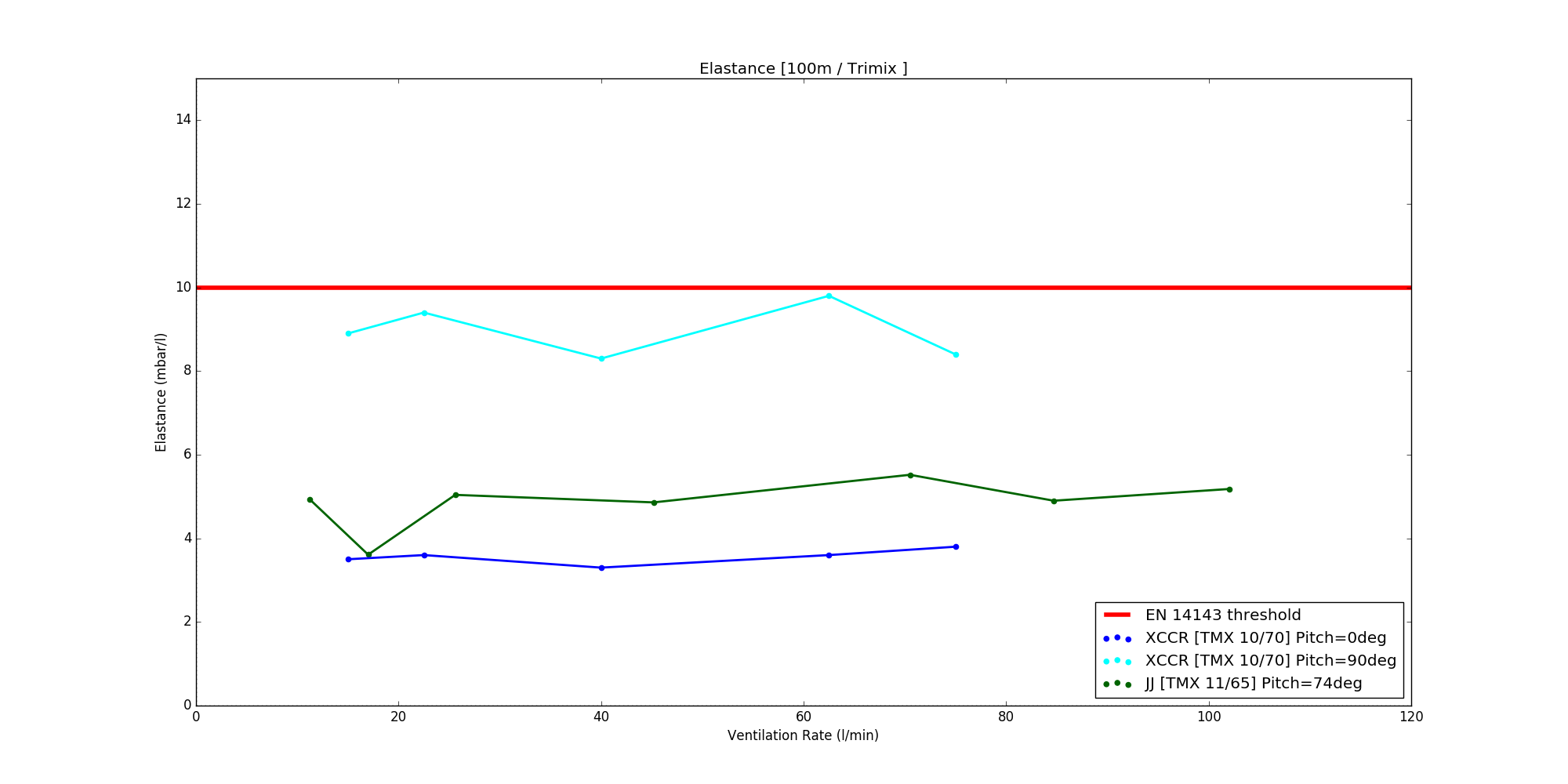PEAK TO PEAK RESPIRATORY PRESSURE

• 40m (Air)

At both pitch angles of 0o and 90o, the X-CCR performs better than the JJ.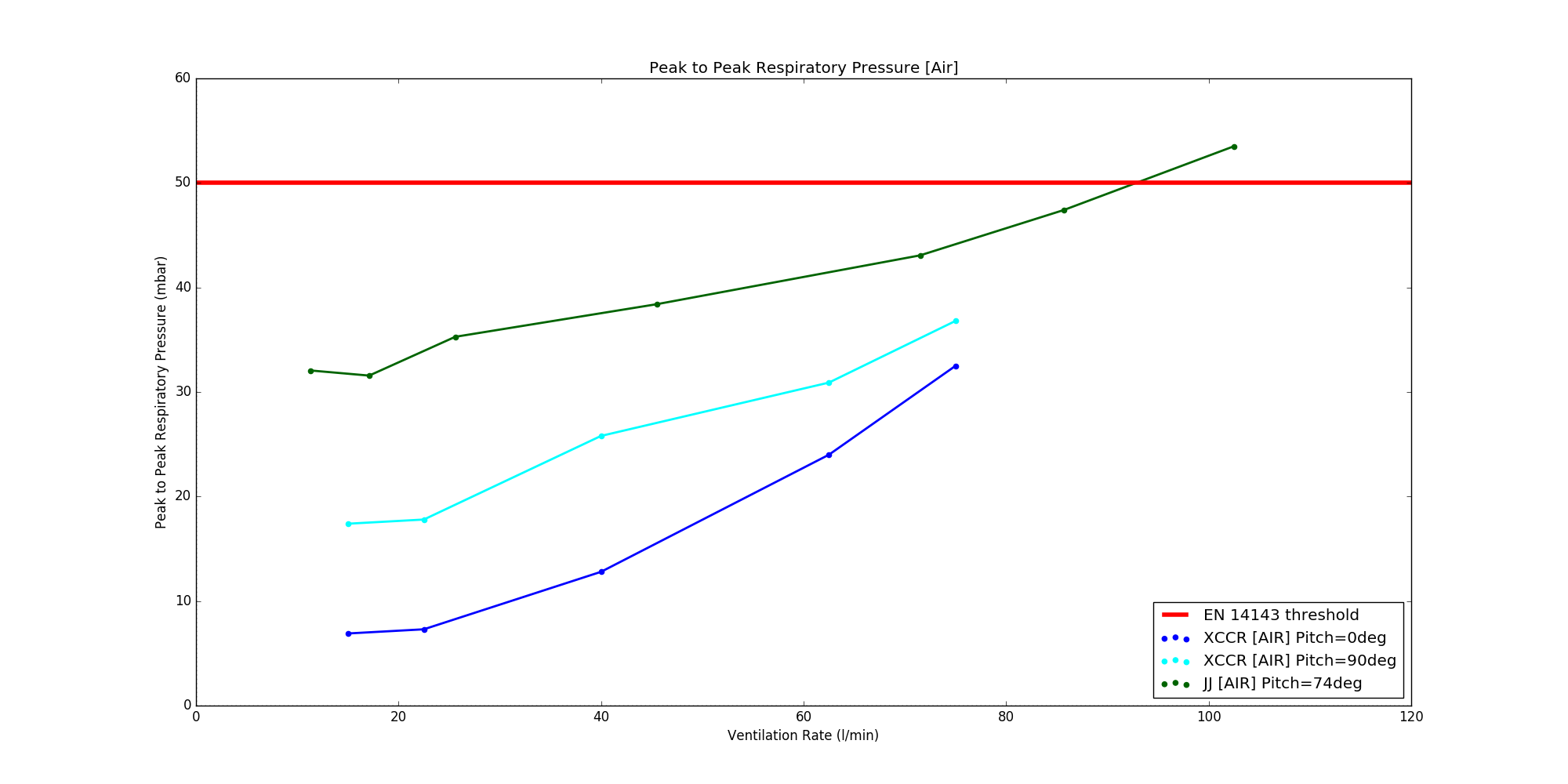• 100m (Trimix)

At pitch angle=0o, the X-CCR performs slightly better than the JJ (pitch angle=74o).
At pitch angle=90o, the X-CCR performs slightly worse than the JJ (pitch angle=74o).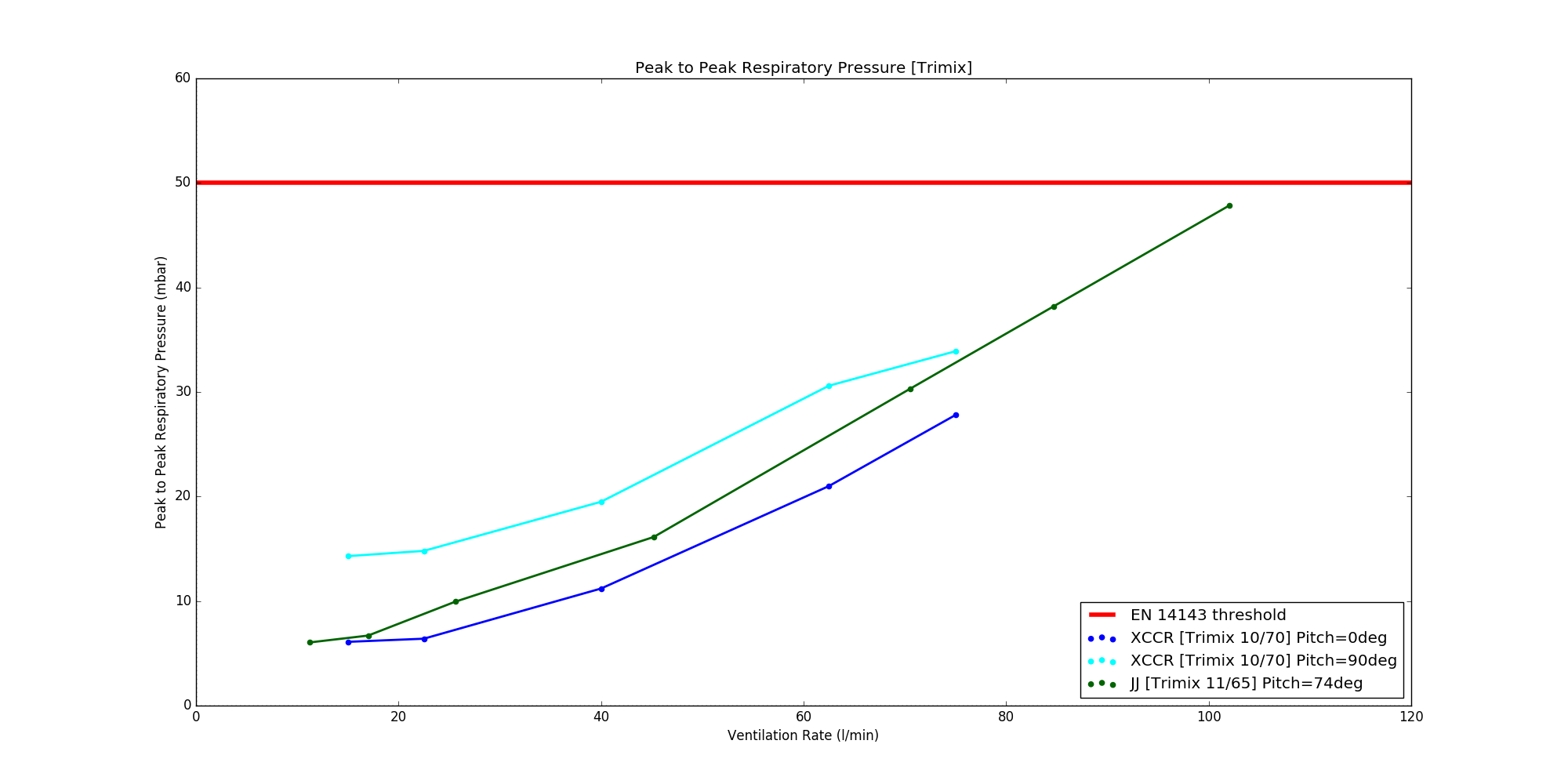SOURCES

JJ-CCR Test Results: